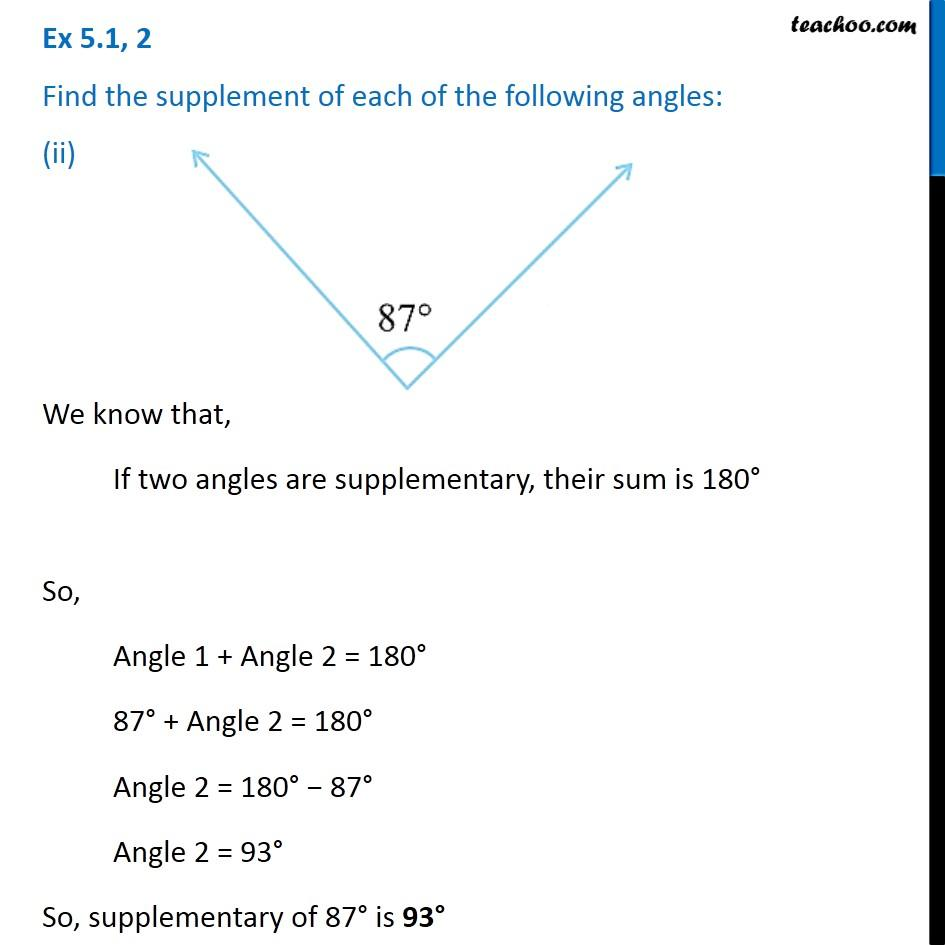Ex 5.1

Chapter 5 Class 7 Lines and Angles
Serial order wiseLearn in your speed, with individual attention - Teachoo Maths 1-on-1 Class

### Transcript

Ex 5.1, 2 Find the supplement of each of the following angles: (ii) We know that, If two angles are supplementary, their sum is 180° So, Angle 1 + Angle 2 = 180° 87° + Angle 2 = 180° Angle 2 = 180° − 87° Angle 2 = 93° So, supplementary of 87° is 93°